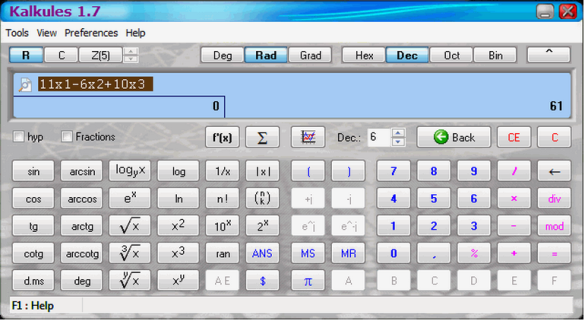# Kalkules Universal Scientific Calculator with Nontraditional Functions

Kalkules is an universal scientific calculator that comes with many nontraditional functions and built-in tools designed to make your calculations easier and faster.

Features:

• Evaluating whole expressions ( ex: 5 + 10 / 3 )[advt]
• Drawing function graphs.
• Calculating with real complex or modulo numbers.
• Calculating in four number systems; binary, octal, decimal, hexadecimal.
• Calculating with fractions ( ex: 5 / 2 + 1 / 3 = 17 / 6 )
• Calculating with polynomials.
• Evaluation history.
• Unlimited count of brackets in the expression.
• Wide range of arithmetic goniometric and hyperbolic functions.
• Using self-named variables eg: radius, volume.
• Inserting some of the predefined constants + ability to define your own constants.
• Inteligent percentage calculations.